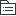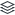# 会计学基础

722
4582

722
|
4582

## 相关模板推荐

•## 会计基础 —— 作品大纲

•1. 会计总论
• 1. 会计的定义
• 会计信息系统论：会计是一个信息系统。
• 会计管理活动论： 会计是对企业经营进行反映和监督的一个活动。
• 2. 会计的职能
• 基本职能：反映和监督
• 扩展职能：分析，预测经济前景等
• 3. 会计的目标
• 决策有用观： 利用会计信息决策
• 受托责任观：股东委托经理人管理公司
• 4. 会计核算方法
• 设置账户
• 复式记账
• 借贷记账法（当前使用）
• 填制和审批凭证
• 登记账簿
• 成本核算
• 财产清查
• 编制会计报表
•2. 会计要素和会计等式
• 1. 会计要素
• 1. 资产
• 含义
• 过去形成
• 由企业拥有或者控制
• 预期会带来经济利益
• 流动性
• 划分： 一年或者一个营业周期
• 流动资产
• 库存现金
• 银行存款
• 应收账款
• 预付账款
• 应收票据
• 原材料
• 库存商品
• 非流动资产
• 长期股权投资
• 固定资产
• 条件
• 为生产或者经营管理而持有
• 寿命超过一个会计年度
• 常见
• 办公场所
• 生产工具
• 无形资产
• 条件
• 无实物形态的非货币资产
• 常见
• 专利权
• 商标权
• 2. 负债
• 含义
• 过去形成
• 将来以债权人所能承受的经济资源清偿
• 负债清偿导致经济利益流出企业
• 流动性
• 划分： 一年或者一个营业周期
• 流动负债
• 短期借款： 一年以下的各种借款
• 应付款项
• 应付票据
• 应付账款
• 应收职工薪酬
• 应交税费
• 应付利息
• 应付股利
• 其他应付款
• 预收账款
• 非流动负债
• 长期借款：一年以上的各种借款
• 应付债券： 企业为筹集长期资金而发行的长期债券
• 长期应付款： 除了长期借款和应付债券以外的长期应付款项
• 3. 所有者权益
• 含义： 股东权益 = 资产 - 负债
• 构成
• 实收资本（一般有限）/股本（股份有限）
• 资本公积
• 资本投入所产生的溢价
• 直接计入所有者权益的利得和损失
• 主要用于转增资本，实收资本/股本
• 盈余公积
• 法定盈余公积： 《公司法》规定必须提取的公积金
• 任意盈余公积： 企业本身要求提取的公积金
• 主要用于弥补亏损，转增资本，分派股利
• 未分配利润
• 4. 收入
• 含义（狭义）
• 日常活动形成（偶发的横财不算）
• 资产增加或者负债减少
• 不包括
• 第三方或者客户代收的款项
• 投资者投资
• 构成（狭义 营业收入）
• 主营业务收入（基本业务收入）
• 其他业务收入（附营业务收入）
• 投资收益（亏钱也正常啊）
• 构成（广义）
• 营业外收入：与经营活动无关的收入
• 5. 费用
• 含义
• 日常发生
• 资产减少或者负债增加
• 不包括
• 向所有者分配利润
• 构成
• 成本
• 特定对象的成本，通常与生产产品和提供劳务有关
• 制造成本（制造业）
• 直接材料
• 人工
• 制造费用
• 费用
• 一种“期间”的费用，与制造生产无关
• 营业税费
• 期间费用
• 销售费用
• 管理费用
• 财务费用
• 贷款利息
• 资产减值损失
• 6. 利润
• 含义
• 一个会计期间
• 收入减去费用的净额
• 所有者权益增加
• 构成
• 营业利润
• 收入
• + 主营业务收入
• + 其他业务收入
• + 投资收益
• + 公允价值变动损益
• 费用
• 成本
• - 主营业务成本
• - 其他业务成本
• 费用
• - 营业税金及附加（流转）
• - 期间费用
• 销售费用
• 管理费用
• 财务费用
• - 资产减值损失
• 利润总额
• + 营业利润
• + 营业外收入
• - 营业外支出
• 净利润
• + 利润总额
• - 所得税
• 2. 会计等式
• 三种形式
• 基本会计等式
• 基本形式：资产 = 负债 + 所有者权益
• 变形形式：资产 - 负债 = 所有者权益
• 静态会计等式，指企业在某一个时点的等式
• 动态会计等式
• 形式： 收入 - 费用 = 利润
• 会计增量等式： 企业在一段期间的经营成果
• 扩展会计等式
• 形式：资产 = 负债 + 所有者权益 + （收入 - 费用）
• 形式2： 资产 = 负债 + 所有者权益 + 利润
• 经济业务对等式的影响
• 基本经济业务员类型
• 资产项目一增一减
• 负债项目一增一减
• 所有者权益一增一减
• 负债增 所有者权益减
• 所有者权益增 负债减
• 资产增负债增
• 资产增所有者增
• 资产减负债减
• 资产减所有者权益减
•3. 会计确认和计量
• 会计核算基础
• 会计假设
• 含义： 对会计工作的理设定
• 时间范围
• 空间范围
• 基本程序
• 计量方法
• 构成
• 会计主体假设 - 会计工作的空间范围
• 持续经营假设 - 会计工作的时间范围， 在可预见的将来企业将持续经营
• 例子： 企业破产了，借款不用还咯，要还的款谁还借
• 会计分期假设
• 按会计年度编制财务报表，季度，月度等
• 货币计量假设
• 采用币值稳定的货币为前提
• 会计信息质量特征（评价标准）
• 可靠性（客观性，真实性）
• 相关性（有用性）
• 可理解性
• 可比性
• 实质重于形式
• 重要性
• 谨慎性
• 及时性
• 会计处理基础
• 收付实现制（现金制/实收实付制）
• 不存在账项调整
• 核算手续简单
• 权责发生制
• 期末进行账项调整
• 例子： 物业费一次性交一年，需要分摊
• 核算手续复杂
•4. 账户与复式记账
• 1. 账户
• 含义
• 对会计要素增减变动进行分类记录，反映的工具
• 是对会计要素的进一步分类
• 设置原则
• 全面反映会计要素内容
• 满足对外报告和内部管理的需求
• 发展与稳定
• 统一性与灵活性
• 简明适用
• 账户复式记账示例
• 原材料
• 2. 会计科目
• 含义
• 会计科目就是账户的名称
• 账户根据会计科目开设
• 区别还是有点的
• 会计科目分类
• 国家有统一总分类科目（一级分类科目/总账科目）
• 二级分类科目
• 明细分类科目/三级分类科目
• 3. 复式记账原理
• 含义
• 任意经济业务必须在两个或者两个以上账户中进行登记
• 需要设置完整的账户体系
• 全面记录经济业务
• 双重记账
• 定期汇总，综合试算
• 4. 借贷记账法
• 记账符号及其双重内涵
• 账户结构
• 资产类账户 + 借方有余额
• 负债 - 贷款有余额
• 所有者权益账户 - 贷方有余额
• 收入类 - 一般无余额
• 费用类账户 + 一般无余额
• 利润类 - 利润为正，贷方有余额，反之相反
• 记账规则
• 有借必有贷，借贷必相等
• 会计分录
• 类似于凭证
• 试算平衡
• 含义
• 通过汇总检查记账错误
• 方式
• 发生额平衡法
• 全部账户借贷平衡
• 余额平衡法
• 全部账户借方余额 = 全部账户贷方余额
• 实际操作
• 编制总账发生额与余额合计试算
• 5. 总分类账户与明细分类账户
• 总账（总分类账/一级账户）
• 仅以货币计量
• 明细账（细分类账户）
• 平行登记
• 同时在总分类账和明细账中登记
• 同内容
• 同方向
•5. 制造业主要经济业务的核算
• 1. 资金筹集业务核算
• 2. 供应过程业务核算
• 3. 生产过程业务核算
• 4. 销售过程业务核算
• 5. 财务成果形成核算
• 6. 财务成果分配核算
•6. 会计凭证
• 含义
• 记录经济业务
• 据以等级账簿
• 书面证明
• 种类
• 原始凭证
• 经济业务发生最初取得
• 种类
• 自制原始凭证
• 一次凭证
• 累计凭证
• 汇总原始凭证
• 外来原始凭证
• 一次凭证
• 记账凭证
• 根据原始凭证归类整理编制
•7. 会计账簿
• 含义
• 以会计凭证为依据
• 序时，连续，系统地记录经济业务
• 作用
• 对凭证资料的系统总结
• 考核企业经营情况的重要依据
• 会计报表的主要资料来源
• 种类（用途）
• 序时账簿（日记账）
• 含义
• 以时间先后顺序记录的账本
• 分类
• 普通日记账（通用日记账簿）
• 特种日记账（必须要有）
• 库存现金日记账
• 银行存款日记账
• 分类账簿
• 总分类账簿
• 一般只有一本
• 明细分类账簿
• 备查账簿（非主要账簿，不一定按借贷法登记）
• 例子
• 采购合同
• 销售合同
• 应收票据
• 租入资产
• 种类（外表）
• 订本式账簿
• 一般固定200页，适用于重要账簿，不便修改
• 活页式账簿
• 适用明细账簿
• 适用于固定资产明细账
• 账簿设置
• 账簿登记
• 错账更正
• 结账
• 对账
•8. 财产清查
• 概述
• 根据账簿记录，查询各项财产的实存数与账面结存是否相符
• 通过财产清查，保证账实相符
• 分类
• 按清查范围
• 全部清查
• 局部清查
• 按清查时间
• 定期清查
• 不定期清查
• 按执行单位
• 内部清查
• 外部清查
• 存货盘存制度
• 永续盘存制（贵重物品）
• 含义
• 也叫账面盘存制，通过等级明细账簿，逐笔登记收入支出，并随时计算结存数量
• 优点
• 及时发现问题
• 缺点
• 工作量大，成本高
• 实地盘存制（非贵重物品）
• 含义
• 也成以存计销制，以期末盘点数为准,倒推出本期消耗数量和期末存货的成本
• 优点
• 工作量少
• 缺点
• 手续不严密。核算不及时
• 银行存款的清查
• 银行存款日记账与银行对账单核对
• 存在未达账项
• 种类
• 企业收款但银行尚未收款入账
• 企业已经付款但银行尚未付款入账
• 银行已经收款入账而企业尚未收款入账
• 银行已经付款入账而企业尚未付款入账
• 解决办法
• 编写银行存款余额调节表
• 余额调节表不是原始凭证，不能填入银行存款日记账中
• 其他资产清查
• 库存现金清查
• 实物资产清查
• 应收款项清查
• 询证核对法
• 清查结果的结果
• 步骤
• 核准金额，查明不符原因
• 调整账簿记录，做到账实相符
• 批准后的账务处理
• 待处理财产损益（盘亏，盘盈）
• 原始凭证记录（账存实存对比表）
• 待处理流动资产损益
• 待处理固定资产损益
• 转销
•9. 最终目标： 会计报表
• 含义
• 对外财务报告
• 作用
• 向利益相关者提供信息
• 落实和考核经济责任履行情况
• 有助于使用者做出经济决策
• 构成
• 会计报表
• 资产负债表
• 利润表
• 现金流量表
• 所有者权益变动表
• 会计报表附注
• 主表中列示的文字说明和明细资料
• 未能在主表中列示项目的说明
• 其他财务报告
• 编制基础和方式不受会计准则约束
• 包括定性信息和非会计信息
• 主要包括
• 管理当局的分析与讨论预测报告
• 物价变动影响报告
• 社会责任报告
• 要求
• 质量要求
• 时间要求
• 6天，15天，60天，4个月
• 财务报告形式要求
• 现金流量表
• 含义
• 一个周期内现金及现金等价物的流入和流出
• 现金
• 库存现金，银行存款，其他货币资金
• 现金等价物
• 交易性金融资产中的短期债权
• 编制基础
• 收付实现制
• 作用
• 提供一个周期内现金流入流出信息
• 评价企业支付能力，偿债能力和周转能力
• 有助于预测企业未来现金流量
• 分析企业收益质量及英雄现金净流量的因素
• 组成内容
• 经营活动
• 投资活动
• 筹资活动
• 现金及现金等价物净增加额
• 期末现金及现金等价物余额
• 所有者权益变动表
• 含义
• 反映所有者权益各项目的增减变动情况
• 作用
• 可提供企业某一会计年度所有者权益各项目的增加，减少及其余额的情况
• 预测未来趋势
• 组成部分
• 上年年末余额
• 本年年初余额
• 本年增加变动金额
• 净利润
• 利得和损失
• 所有者投资
• 利润分配
• 本年年末余额
• 报表附注
• 含义
• 对主表中列示的项目的文字说明或者明细资料
• 内容
• 企业基本情况
• 财务报表的编制基础
• 遵循企业会计准则的声明
• 重要会计政策和估计
• 。。。
• 子主题
•10. 主题